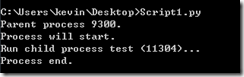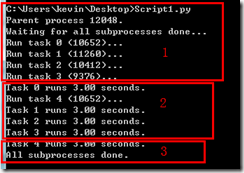Queue是python中的標準庫，可以直接import 引用，之前學習的時候有聽過著名的“先吃先拉”與“後吃先吐”，其實就是這裡說的佇列，佇列的構造的時候可以定義它的容量，別吃撐了，吃多了，就會報錯,構造的時候不寫或者寫個小於1的數則表示無限多

import Queue

q = Queue.Queue(10)

q.put(‘yang’)

q.put(4)

q.put([‘yan’,’xing’])

>>> q.get()
'yang'
>>> q.get()

>>> q.get()
['yan', 'xing']
>>>

get_nowait()方法，這種方法在向一個空佇列取值的時候會拋一個Empty異常

Queue.qsize() 返回佇列的大小
Queue.empty() 如果佇列為空，返回True,反之False
Queue.full() 如果佇列滿了，返回True,反之False
Queue.get([block[, timeout]]) 獲取佇列，timeout等待時間
Queue.get_nowait() 相當Queue.get(False)

Queue.put_nowait(item) 相當Queue.put(item, False)

from multiprocessing import Process

p = Process(target=fun,args=(args))

from multiprocessing import Process
import os

# 子程序要執行的程式碼
defrun_proc(name):
print 'Run child process %s (%s)...' % (name, os.getpid())

if __name__=='__main__':
print 'Parent process %s.' % os.getpid()
p = Process(target=run_proc, args=('test',))
print 'Process will start.'
p.start()
p.join()
print 'Process end.'from multiprocessing import Pool
from multiprocessing import Pool
import os, time

print 'Run task %s (%s)...' % (name, os.getpid())
start = time.time()
time.sleep(3)
end = time.time()
print 'Task %s runs %0.2f seconds.' % (name, (end - start))

if __name__=='__main__':
print 'Parent process %s.' % os.getpid()
p = Pool()
for i in range(5):
print 'Waiting for all subprocesses done...'
p.close()
p.join()
print 'All subprocesses done.'

pool建立子程序的方法與Process不同，是通過#coding:gbk

from multiprocessing import Process, Queue
import os, time, random

# 寫資料程序執行的程式碼:
defwrite(q):
for value in ['A', 'B', 'C']:
print 'Put %s to queue...' % value
q.put(value)
time.sleep(random.random())

# 讀資料程序執行的程式碼:
while True:
if not q.empty():
value = q.get(True)
print 'Get %s from queue.' % value
time.sleep(random.random())
else:
break

if __name__=='__main__':
# 父程序建立Queue，並傳給各個子程序：
q = Queue()
pw = Process(target=write, args=(q,))
# 啟動子程序pw，寫入:
pw.start()
# 等待pw結束:
pw.join()
# 啟動子程序pr，讀取:
pr.start()
pr.join()
# pr程序裡是死迴圈，無法等待其結束，只能強行終止:
print
print '所有資料都寫入並且讀完'

if __name__=='__main__':
# 父程序建立Queue，並傳給各個子程序：
q = Queue()
p = Pool()
pw = p.apply_async(write,args=(q,))
p.close()
p.join()

print
print '所有資料都寫入並且讀完'

RuntimeError: Queue objects should only be shared between processes through inheritance

if __name__=='__main__':
manager = multiprocessing.Manager()
# 父程序建立Queue，並傳給各個子程序：
q = manager.Queue()
p = Pool()
pw = p.apply_async(write,args=(q,))
time.sleep(0.5)
p.close()
p.join()

print
print '所有資料都寫入並且讀完'

#coding:gbk

from multiprocessing import Process,Queue,Pool
import multiprocessing
import os, time, random

# 寫資料程序執行的程式碼:
defwrite(q,lock):
lock.acquire() #加上鎖
for value in ['A', 'B', 'C']:
print 'Put %s to queue...' % value
q.put(value)
lock.release() #釋放鎖

# 讀資料程序執行的程式碼:
while True:
if not q.empty():
value = q.get(False)
print 'Get %s from queue.' % value
time.sleep(random.random())
else:
break

if __name__=='__main__':
manager = multiprocessing.Manager()
# 父程序建立Queue，並傳給各個子程序：
q = manager.Queue()
lock = manager.Lock() #初始化一把鎖
p = Pool()
pw = p.apply_async(write,args=(q,lock))